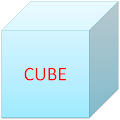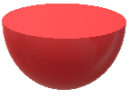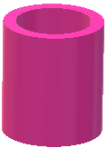### Common Errors in Secondary Mathematics

Common Errors Committed  by the  Students  in Secondary Mathematics   Errors  that students often make in doing secondary mathematics  during their practice and during the examinations  and their remedial measures are well explained here stp by step.  Some Common Errors in Mathematics

### Lesson Plan Math Class X (Ch-13) | Surface Area and Volume

E- LESSON PLAN   SUBJECT MATHEMATICS    CLASS 10

lesson plan for maths class 10 cbse lesson plans for mathematics teachers,  Method to write lesson plan for maths class 4, lesson plan for maths class 12 rational numbers, lesson plan for mathematics grade 10, lesson plan for maths in B.Ed.

 TEACHER'S NAME  :   DINESH KUMAR SCHOOL :  RMB DAV CENTENARY PUBLIC SCHOOL NAWANSHAHR SUBJECT   :   MATHEMATICS CLASS                  :   X  STANDARDBOARD                 :  CBSE LESSON TOPIC / TITLE   : CHAPTER 13:  SURFACE AREA & VOLUME ESTIMATED DURATION: This topic is divided into seven modules and are completed in twelve class meetings.

PRE- REQUISITE KNOWLEDGE:-
TEACHING AIDS:-

Green Board, Chalk,  Duster, Charts, solid figures, projector, laptop  etc.

METHODOLOGY:-

Lecture method, Demonstration and Learning by doing.

LEARNING OBJECTIVES:
• Introduction of different types of solid figures and their comparison with the plane figures.
• Curved surface area, total surface area and volume of different solid figures.
• Surface area and volume of combination of solid figures.
• Method of converting one type of solid figure into another.
• Other mixed problems (combination of not more than two solid figures be taken)
LEARNING  OUTCOMES:

After studying this lesson students should know
• Different shapes of solid figures.
• All formulas and all important concepts related to the surface area of solid figures.
• All formulas and all important concepts related to the volume of solid figures.
• Solving the problems related to the solid figures.
• Students should also know the method of implementation of these formulas in simple and complex problems.
RESOURCES
NCERT Text book of Mathematics
NCERT Exemplar Text Book
RESOURCE CENTRE at cbsemathematics.com

KEY WORDS :
Surface area, volume, cube, cuboid, cylinder, cone, sphere, hemi-sphere, frustum of cone.
PROCEDURE
In this lesson, learners will calculate the surface areas and the volumes of the combination of solids. They will also learn that when a solid is converted to another solid or multiple solids, either of the same or different shapes, the surface area changes but the volume remains constant. Moreover, they will also be taught to calculate the surface area and the volume of the frustum of a cone.

Start the session by checking their previous knowledge, by asking the questions of surface area and volumes of solid figures. After this explain the topic to the students as follows.

Teacher-Student Activities A. Warm-up Session

Begin the lesson by recalling the prior knowledge of students on the following topics:

• Surface area and volumes of cube and cuboid.
• Surface area and volume of cylinder and cone.
• Surface area and volume of  sphere and hemi- sphere.

Recall the formulae to calculate the surface areas and the volumes of different basic solids.

Then, show different objects (or their images) that are made by the combination of different solids.Teacher’s Explanation

Now teacher will provide complete explanations of all the formulas of the solid figures and ask the students to learn these formulas. Following solid figures need to be discussed here.
Surface area and volumes of cube and cuboid.

• Surface area and volume of cylinder.
• Surface area and volume of cone.
• Surface area and volume of sphere and hemi- sphere.
• Surface area and volume of Frustum of cone.

Combination of solid Figure.

Here we combine two or more solid figures and then find the surface area and volumes. Teacher will explain the method of finding the surface area and volume in the combination form.Surface Areas and Volumes: Clay Activity

In this activity, students will calculate the surface areas and the volumes of the combination of solids.

In this activity, students with the help of clay make some solid figures and  will calculate the change, if any, in the surface area and the volume of a solid when it is converted into different solid or multiple solids, either of the same or different shapes.Explanation of the Activity

Divide the class into two groups. Bring some modeling clay to the classroom. Ask a group to shape the clay in any of the basic solids, such as cube, cuboid, cylinder and sphere. They need to take the required measurements and calculate the surface area and the volume of the solid that they have made. Now, the other group should break the solid and make numerous smaller solids from the clay. Then, they need to take the required measurements and calculate the surface area and the volume of the smaller shapes. Thereafter, ask both groups to analysis the relation between the surface area and the volume of the original solid and the new solids. Conduct a similar activity to prove that when the shape of a solid object is changed, its surface area changes but the volume remains constant. For this activity, change the original basic solid into the basic solid of a different shape.For example, change a cube into a cylinder and compare the surface area and the volume of the cube with the cylinder.

Practice Work

Now teacher will explain the method of finding surface area and volumes by taking some examples from NCERT book. Teacher will also provide some questions to the  students for practice

Practice with combination of solid figures

Teacher will also explain  some other mixed problems [for the problems of combination of solid figures, not more than two solids be taken in combination] and should provide  sufficient number of problems to the students for practice.

STUDENTS DELIVERABLES:-
• Review questions given by the teacher.
• Students should prepare the presentation on the formulas of surface area and volumes of different solid figures.
• Solve NCERT problems with examples.
• Solve assignment given by the teacher.
EXTENDED LEARNING:-

Students can extend their learning through the RESOURCE CENTRE and can find more valuable and interesting concepts on mathematics at  cbsemathematics.com

ASSESSMENT TECHNIQUES:-
Assignment sheet will be given as home work at the end of the topic. Separate sheets which will include questions of logical thinking and Higher order thinking skills will be given to the above average students.
Class Test , Oral Test , worksheet and Assignments can be made the part of assessment.
Re-test(s) will be conducted on the basis of the performance of the students in the test.

FEEDBACK:
All those students who performed well will be appreciated positively, and all those who can not perform up to the mark will again be given some important tips, guidance and  positive motivation to go through the topic again and then re-evaluated again. If possible then provision of remedial classes can be made for batter results.

🙏

1.